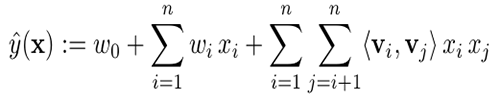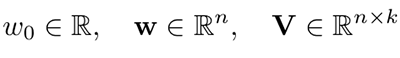# Extending Amazon SageMaker factorization machines algorithm to predict top x recommendations

Amazon SageMaker gives you the flexibility that you need to address sophisticated business problems with your machine learning workloads. Built-in algorithms help you get started quickly.  In this blog post we’ll outline how you can extend the built-in factorization machines algorithm to predict top x recommendations.

This approach is ideal when you want to generate a set number of recommendations for users in a batch fashion. For example, you can use this approach to generate the top 20 products that a user is likely to buy from a large set of users and product purchase information. You can then store the recommendations in a database for further use, such as dashboard display or personalized email marketing. You can also automate the steps outlined in this blog for periodic retraining and prediction using AWS Batch or AWS Step Functions.

A factorization machine is a general-purpose supervised learning algorithm that you can use for both classification and regression tasks. This algorithm was designed as an engine for recommendation systems. It extends the collaborative filtering approach by learning a quadratic function over the features while restricting second order coefficients to a low rank structure. This restriction is well-suited for large and sparse data because it avoids overfitting and is highly scalable, so that a typical recommendation problem with millions of input features will have millions of parameters rather than trillions

The model equation for factorization machines is defined as:Model parameters to be estimated are:where, n is the input size and k is the size of the latent space. These estimated model parameters are used to extend the model.

# Model extension

The Amazon SageMaker factorization machines algorithm allows you to predict a score for a pair, such as user, item, based on how well the pair matches. When you apply a recommendation model, you often want to provide a user as input and receive a list of the top x items that best match the user’s preferences. When the number of items is moderate, you can do this by querying the model for user, item for all possible items. However, this approach doesn’t scale well when the number of items is large. In this scenario, you can use the Amazon SageMaker k-nearest neighbors (k-NN) algorithm to speed up top x prediction tasks.

The following diagram provides a high-level overview of the steps covered in this blog post, which include building a factorization machines model, repackaging model data, fitting a k-NN model, and producing top x predictions.You can also download a companion Jupyter notebook to follow along. Each of the following sections corresponds to a section in the notebook so that you can run the code for each step as you read.

# Step 1: Building a factorization machines model

See Part 1 of the companion Jupyter notebook for steps to build a factorization machines model. To learn more about building factorization machines models, see the Factorization Machines documentation.

# Step 2: Repackaging model data

The Amazon SageMaker factorization machines algorithm leverages Apache MXNet deep learning framework. In this section, we’ll cover how to repackage the model data using MXNet.

## Extract the factorization machines model

First, you’ll download the factorization model, and then you’ll decompress it for constructing an MXNet object. The main purpose of the MXNet object is to extract the model data.

``````#Download FM model
os.system('aws s3 cp '+{Model location} + './')

#Extract files from the model. Note: the companion notebook outlines the extraction steps.``````

## Extract model data

The input to a factorization machines model is a list of vectors xu + xi representing user u and item i coupled with a label, such as a user rating for a movie. The resulting input matrix will include sparse one-hot encoded values for users, items, and any additional features you may want to add.

The factorization machines model output consists of three N-dimensional arrays (ndarrays):

• V – a (N x k) matrix, where:
• k is the dimension of the latent space
• N is the total count of users and items
• w – an N-dimensional vector
• b – a single number: the bias term

Complete the steps below to extract the model output from the MXNet object.

``````#Extract model data
m = mx.module.Module.load('./model', 0, False, label_names=['out_label'])
V = m._arg_params['v'].asnumpy()
w = m._arg_params['w1_weight'].asnumpy()
b = m._arg_params['w0_weight'].asnumpy()
``````

## Prepare data to build a k-NN model

Now you can repackage the model data extracted from the factorization machines model to build a k-NN model. This process will create two datasets:

• Item latent matrix – for building the k-NN model
• User latent matrix – for inference
``````nb_users = <num users>
nb_movies = <num items>

# item latent matrix - concat(V[i], w[i]).
knn_item_matrix = np.concatenate((V[nb_users:], w[nb_users:]), axis=1)
knn_train_label = np.arange(1,nb_movies+1)

#user latent matrix - concat (V[u], 1)
ones = np.ones(nb_users).reshape((nb_users, 1))
knn_user_matrix = np.concatenate((V[:nb_users], ones), axis=1)
``````

# Step 3: Fitting a k-NN model

Now you can upload the k-NN model input data to Amazon S3, create a k-NN model, and save it so that it can be used in Amazon SageMaker. The model will also come in handy for calling batch transforms, as described in the following steps.

The k-NN model uses the default index_type (faiss.Flat). This model is precise, but it can be slow for large datasets. In such cases, you may want to use a different index_type parameter for an approximate but faster answer. For more information about index types, see either the k-NN documentation or this Amazon Sagemaker Examples notebook.

``````#upload data
knn_train_data_path = writeDatasetToProtobuf(knn_item_matrix, bucket, knn_prefix, train_key, "dense", knn_train_label)

# set up the estimator
nb_recommendations = 100
knn = sagemaker.estimator.Estimator(get_image_uri(boto3.Session().region_name, "knn"),
get_execution_role(),
train_instance_count=1,
train_instance_type=instance_type,
output_path=knn_output_prefix,
sagemaker_session=sagemaker.Session())

#set up hyperparameters
knn.set_hyperparameters(feature_dim=knn_item_matrix.shape, k=nb_recommendations, index_metric="INNER_PRODUCT", predictor_type='classifier', sample_size=nb_movies)
fit_input = {'train': knn_train_data_path}
knn.fit(fit_input)
knn_model_name =  knn.latest_training_job.job_name
print "created model: ", knn_model_name

# save the model so that you can reference it in the next step during batch inference
sm = boto3.client(service_name='sagemaker')
primary_container = {
'Image': knn.image_name,
'ModelDataUrl': knn.model_data,
}
knn_model = sm.create_model(
ModelName = knn.latest_training_job.job_name,
ExecutionRoleArn = knn.role,
PrimaryContainer = primary_container)
``````

# Step 4: Predicting Top x recommendations for all users

The Amazon SageMaker batch transform feature lets you generate batch predictions at scale. For this example, you’ll start by uploading user inference input to Amazon S3, and then you’ll trigger a batch transform.

``````#upload inference data to S3
knn_batch_data_path = writeDatasetToProtobuf(knn_user_matrix, bucket, knn_prefix, train_key, "dense")
print "Batch inference data path: ",knn_batch_data_path

# Initialize the transformer object
transformer =sagemaker.transformer.Transformer(
base_transform_job_name="knn",
model_name=knn_model_name,
instance_count=1,
instance_type=instance_type,
output_path=knn_output_prefix,
accept="application/jsonlines; verbose=true"
)

# Start a transform job:
transformer.transform(knn_batch_data_path, content_type='application/x-recordio-protobuf')
transformer.wait()

``````

The resulting output file will contain predictions for all users. Each line item in the output file is a JSON line containing item IDs and distances for a specific user.

Here’s a sample output for a user. You can store the recommended movie IDs to your database for further use.

``````Recommended movie IDs for user #1 : [509, 1007, 96, 210, 208, 505, 268, 429, 182, 189, 57, 132, 482, 165, 615, 527, 196, 269, 528, 83, 176, 166, 194, 520, 661, 246, 180, 659, 496, 173, 9, 435, 474, 192, 493, 48, 211, 656, 489, 181, 251, 124, 89, 510, 22, 183, 316, 185, 197, 23, 170, 168, 963, 190, 1039, 56, 79, 136, 519, 651, 484, 275, 654, 641, 523, 478, 302, 223, 313, 187, 1142, 134, 100, 498, 272, 285, 191, 515, 408, 178, 199, 114, 480, 603, 172, 169, 174, 427, 513, 657, 318, 357, 511, 12, 50, 127, 479, 98, 64, 483]

Movie distances for user #1 : [1.8703, 1.8852, 1.8933, 1.905, 1.9166, 1.9185, 1.9206, 1.9239, 1.928, 1.9304, 1.9411, 1.9452, 1.947, 1.9528, 1.963, 1.975, 1.9985, 2.0117, 2.0205, 2.0211, 2.0227, 2.0583, 2.0959, 2.0986, 2.1064, 2.1126, 2.1157, 2.119, 2.1208, 2.124, 2.1349, 2.1356, 2.1413, 2.1423, 2.1521, 2.1577, 2.1618, 2.176, 2.1819, 2.1879, 2.1925, 2.2463, 2.2565, 2.2654, 2.2979, 2.3289, 2.3366, 2.3398, 2.3617, 2.3654, 2.3855, 2.386, 2.3867, 2.4198, 2.4431, 2.46, 2.462, 2.4643, 2.4729, 2.4959, 2.5334, 2.5359, 2.5362, 2.542, 2.5428, 2.5934, 2.5953, 2.598, 2.6575, 2.6735, 2.6879, 2.7038, 2.7259, 2.7432, 2.8112, 2.8707, 2.871, 2.9378, 2.9728, 3.0175, 3.0231, 3.0254, 3.0259, 3.0325, 3.0414, 3.1033, 3.2729, 3.3406, 3.392, 3.3982, 3.4196, 3.4452, 3.4684, 3.4743, 3.6265, 3.7013, 3.7711, 3.7736, 3.8898, 4.0698]``````

# Multiple features and categories scenario

The framework in this blog applies to a scenario with user and item IDs. However, your data may include additional information, such as user and item features. For example, you might know the user’s age, zip code, or gender. For the item, you might have a category, a movie genre, or important keywords from a text description. In these multiple-feature and category scenarios, you can use the following to extract user and item vectors:

• encode xi with both the users and user features:
ai =concat(VT · xi , wT · xi)
• encode xu with items and item features:
au =concat(VT · xu, 1)

Then use ai to build the k-NN model and au for inference.

# Conclusion

Amazon SageMaker gives developers and data scientists the flexibility to build, train, and deploy machine learning models quickly. Using the framework outlined above, you can build a recommendation system for predicting the top x recommendations for users in a batch fashion and cache the output in a database. In some cases you may need to apply further filtering on predictions or filter out some of the predictions based on user responses over the time. This framework is flexible enough to modify for such use cases.Zohar Karnin is a Principal Scientist in Amazon AI. His research interests are in the areas of large scale and online machine learning algorithms. He develops infinitely scalable machine learning algorithms for Amazon SageMaker.Rama Thamman is a Sr. Solution Architect with the Strategic Accounts team. He works with customers to build scalable cloud and machine learning solutions on AWS.